Amino Acids

 The Amino Acids Zwitterions The Amino Acids Used to Synthesize Proteins The Acid-Base Chemistry of the Amino Acids

The Amino Acids

Proteins are formed by polymerizing monomers that are known as amino acids because they contain an amine (-NH2) and a carboxylic acid (-CO2H) functional group. With the exception of the amino acid proline, which is a secondary amine, the amino acids used to synthesize proteins are primary amines with the following generic formula.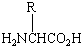An amino acid

These compounds are known as a-amino acids because the -NH2 group is on the carbon atom next to the -CO2H group, the so-called carbon atom of the carboxylic acid.Zwitterions

The chemistry of amino acids is complicated by the fact that the -NH2 group is a base and the -CO2H group is an acid. In aqueous solution, an H+ ion is therefore transferred from one end of the molecule to the other to form a zwitterion (from the German meaning mongrel ion, or hybrid ion).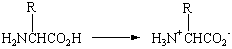Zwitterions are simultaneously electrically charged and electrically neutral. They contain positive and negative charges, but the net charge on the molecule is zero.The Amino Acids Used to Synthesize Proteins

More than 300 amino acids are listed in the Practical Handbook of Biochemistry and Molecular Biology, but only the twenty amino acids in the table below are used to synthesize proteins. Most of these amino acids differ only in the nature of the R substituent. The standard amino acids are therefore classified on the basis of these R groups. Amino acids with nonpolar substituents are said to be hydrophobic (water-hating). Amino acids with polar R groups that form hydrogen bonds to water are classified as hydrophilic (water-loving). The remaining amino acids have substituents that carry either negative or positive charges in aqueous solution at neutral pH and are therefore strongly hydrophilic.

The 20 Standard Amino Acids

 NAME STRUCTURE (AT NEUTRAL pH) Nonpolar (Hydrophobic) R Groups Glycine (Gly)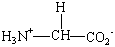Alanine (Ala)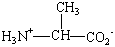Valine (Val)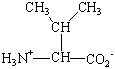Leucine (Leu)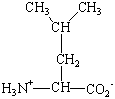Isoleucine (Ile)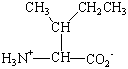Proline (Pro)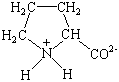Methionine (Met)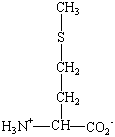Phenylalanine (Phe)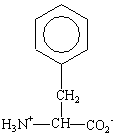Tryptophan (Trp)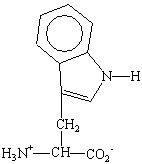Polar (Hydrophilic) R Groups Serine (ser)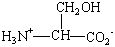Threonine (Thr)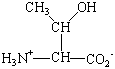Tyrosine (Tyr)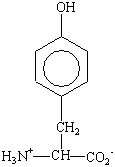Cysteine (Cys)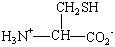Asparagine (Asn)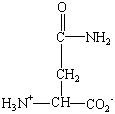Glutamine (Gln)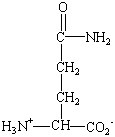Negatively Charged R Groups Aspartic acid (Asp)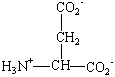Glutamic acid (Glu)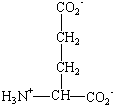Positively Charged R Groups Lysine (Lys)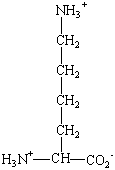Arginine (Arg)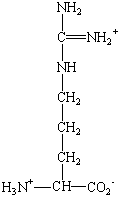Histidine (His)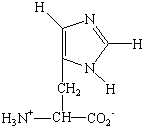Practice Problem 1:Use the structures of the following amino acids in the table of standard amino acids to classify these compounds as either nonpolar/hydrophobic, polar/hydrophilic, negatively charged/hydrophilic, or positively charged/hydrophilic. (a) Valine: R = -CH(CH3)2 (b) Serine: R = -CH2OH (c) Aspartic acid: R = -CH2CO2- (d) Lysine: R = -(CH2)4NH3+ Click here to check your answer to Practice Problem 1Amino Acids as Stereoisomers

With the exception of glycine, the common amino acids all contain at least one chiral carbon atom. These amino acids therefore exist as pairs of stereoisomers. The structures of the D and L isomers of alanine are shown in the figure below. Although D amino acids can be found in nature, only the L isomers are used to form proteins. The D isomers are most often found attached to the cell walls of bacteria and in antibiotics that attack bacteria. The presence of these D isomers protects the bacteria from enzymes the host organism uses to protect itself from bacterial infection by hydrolyzing the proteins in the bacterial cell wall.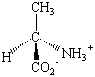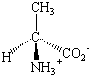D-Alanine L-Alanine

A few biologically important derivatives of the standard amino acids are shown in the figure below. Anyone who has used an "anti-histamine" to alleviate the symptoms of exposure to an allergen can appreciate the role that histaminea decarboxylated derivative of histidineplays in mediating the body's response to allergic reactions. L-DOPA, which is a derivative of tyrosine, has been used to treat Parkinson's disease. This compound received notoriety a few years ago in the film Awakening, which documented it's use as a treatment for other neurological disorders. Thyroxine, which is an iodinated ether of tyrosine, is a hormone that acts on the thyroid gland to stimulate the rate of metabolism.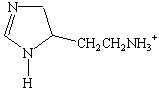Histamine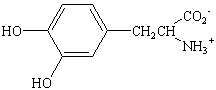L-DOPA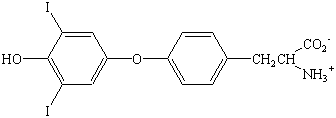ThyroxineThe Acid-Base Chemistry of the Amino Acids

Acetic acid and ammonia often play an important role in the discussion of the chemistry of acids and bases. One of these compounds is a weak acid; the other is a weak base.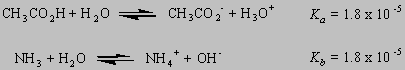Thus, it is not surprising that an H+ ion is transferred from one end of the molecule to the other when an amino acid dissolves in water.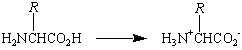The zwitterion is the dominant species in aqueous solutions at physiological pH (pH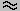7). The zwitterion can undergo acid-base reactions, howeer, if we add either a strong acid or a strong base to the solution.

Imagine what would happen if we add a strong acid to a neutral solution of an amino acid in water. In the presence of a strong acid, the -CO2- end of this molecule picks up an H+ ion to form a molecule with a net positive charge.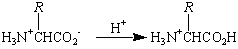In the presence of a strong base, the -NH3+ end of the molecule loses an H+ ion to form a molecule with a net negative charge.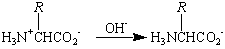The figure below shows what happens to the pH of an acidic solution of glycine when this amino acid is titrated with a strong base, such as NaOH.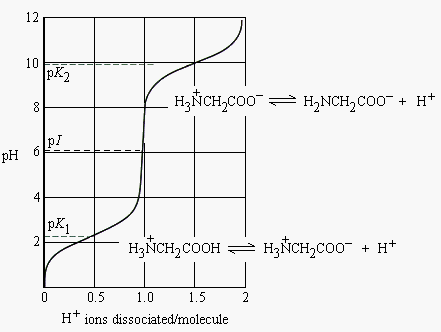In order to understand this titration curve, let's start with the equation that describes the acid-dissociation equilibrium constant expression for an acid, HA.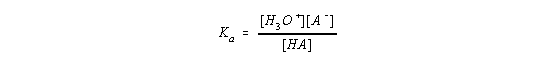Let's now rearrange the Ka expression,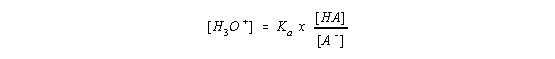take the log to the base 10 of both sides of this equation,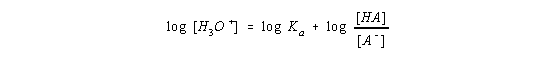and then multiply both sides of the equation by -1.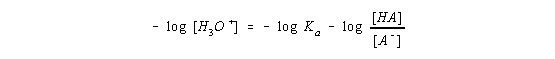By definition, the term on the left side of this equation is the pH of the solution and the first term on the right side is the pKa of the acid.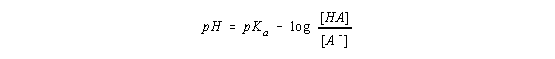The negative sign on this right side of this equation is often viewed as "inconvenient." The derivation therefore continues by taking advantage of the following feature of logarithmic mathematics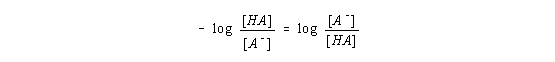to give the following form of this equation.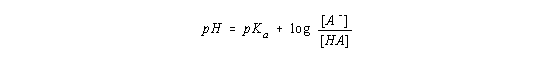This equation is known as the Henderson-Hasselbach equation, and it can be used to calculate the pH of the solution at any point in the titration curve.

The following occurs as we go from left to right across this titration curve.

• The pH initially increases as we add base to the solution because the base deprotonates some of the positively charged H3N+CH2CO2H ions that were present in the strongly acidic solution.
• The pH then levels off because we form a buffer solution in which we have reasonable concentrations of both an acid, H3N+CH2CO2H, and its conjugate base, H3N+CH2CO2-.
• When virtually all of the H3N+CH2CO2H molecules have been deprotonated, we no longer have a buffer solution and the pH rises rapidly when more NaOH is added to the solution.
• The pH then levels off as some of the neutral H3N+CH2CO2- molecules lose protons to form negatively charged H2NCH2CO2- ions. When these ions are formed, we once again get a buffer solution in which the pH remains relatively constant until essentially all of the H3N+CH2CO2H molecules have been converted into H2NCH2CO2- ions.
• At this point, the pH rises rapidly until it reaches the value observed for a strong base.

The pH titration curve tells us the volume of base required to titrate the positively charged H3N+CH2CO2H molecule to the H3N+CH2CO2- zwitterion. If we only add half as much base, only half of the positive ions would be titrated to zwitterions. In other words, the concentration of the H3N+CH2CO2H and H3N+CH2CO2- ions would be the same. Or, using the symbolism in the Henderson-Hasselbach equation:

[HA] = [A-]

Because the concentrations of these ions is the same, the logarithm of the ratio of their concentrations is zero.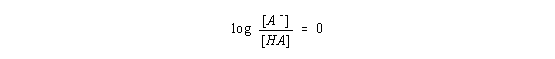Thus, at this particular point in the titration curve, the Henderson-Hasselbach equation gives the following equality.

pH = pKa

We can therefore determine the pKa of an acid by measuring the pH of a solution in which the acid has been half-titrated.

Because there are two titratable groups in glycine, we get two points at which the amino acid is half-titrated. The first occurs when half of the positive H3N+CH2CO2H molecules have been converted to neutral H3N+CH2CO2- ions. The second occurs when half of the H3N+CH2CO2- zwitterions have been converted to negatively charged H2NCH2CO2- ions.

The following results are obtained when this technique is applied to glycine.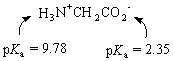Let's compare these values with the pKa's of acetic acid and the ammonium ion.

 CH3CO2H pKa = 4.74 NH4+ pKa = 9.24

The acid/base properties of the a-amino group in an amino acid are very similar to the properties of ammonia and the ammonium ion. The a-amine, however, has a significant effect on the acidity of the carboxylic acid. The -amine increases the value of Ka for the carboxylic acid by a factor of about 100.

The inductive effect of the a-amine can only be felt at the a-CO2H group. If we look at the chemistry of glutamic acid, for example, the a-CO2H group on the R substituent has an acidity that is close to that of acetic acid.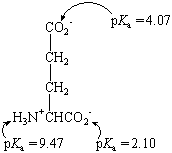When we titrate an amino acid from the low end of the pH scale (pH1) to the high end (pH13), we start with an ion that has a net positive charge and end up with an ion that has a net negative charge.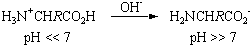Somewhere between these extremes, we have to find a situation in which the vast majority of the amino acids are present as the zwitterionwith no net electric charge. This point is called the isoelectric point (pI) of the amino acid.

For simple amino acids, in which the R group doesn't contain any titratable groups, the isoelectric point can be calculated by averaging the pKa values for the a-carboxylic acid and a-amino groups. Glycine, for example, has a pI of about 6.

pI = 2.35 + 9.78 = 6.1
2

At pH6, more than 99.98% of the glycine molecules in this solution are present as the neutral H3N+CH2CO2H zwitterion.

When calculating the pI of an amino acid that has a titratable group on the R side chain, it is useful to start by writing the structure of the amino acid at physiological pH (pH7). Lysine, for example, could be represented by the following diagram.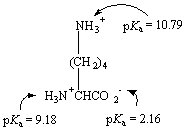At physiological pH, lysine has a net positive charge. Thus, we have to increase the pH of the solution to remove positive charge in order to reach the isoelectric point. The pI for lysine is simply the average of the pKa's of the two -NH3+ groups.

pI = 9.18 + 10.7910.0
2

At this pH, all of the carboxylic acid groups are present as -CO2- ions and the total population of the -NH3+ groups is equal to one. Thus, the net charge on the molecule at this pH is zero.

If we apply the same technique to the pKa data for glutamic acid, given above, we get a pI of about 3.1. The three amino acids in this section therefore have very different pI values.

 Glutamic acid (R = -CH2CH2CO2-): pI = 3.1 Glycine (R = -H): pI = 6.1 Lysine (R = -CH2CH2CH2CH2NH3+): pI = 10.0

Thus, it isn't surprising that a common technique for separating amino acids (or the proteins they form) involves placing a mixture in the center of a gel and then applying a strong voltage across this gel. This technique, which is known as gel electrophoresis, is based on the fact that amino acids or proteins that carry a net positive charge at the pH at which the separation is done will move toward the negative electrode, whereas those with a net negative charge will move toward the positive electrode.